# Passing a function reporter with input slot twice

Hi,
I wrote a procedure wich draws a parameterized function which is passed to this procedure: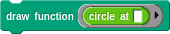The parameterized functions (in this case "circle") accept inputs between 0 and 1 and report a single point as list (x,y). The draw procedure draws all points by running from t=0 to t=1 in steps of e.g. 0.01

Now I want to write a morph procedure, which animates a morphing from one function to another function: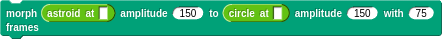The morph procedure should use the draw procedure above to draw a linear combination of the two functions passed to it. I tried something like this inside the morph procedure: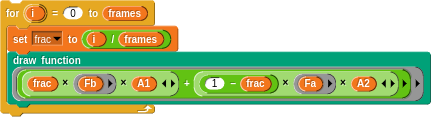But it doesn't work (no error, but nothing is drawn).

Fa and Fb are the parameterized functions which are passed to the morph procedure.
Is it possible to pass Fa and Fb (resp. the linear combination of them) to the draw procedure so that it handles it as a reporter function with empty input slots?

Karl

Calculation involving lambdas (function/ ringified expression / unevaluated parameters) does not create another lambda (rather NaN).
The simplest form to get desired result is to explicity call the embedded function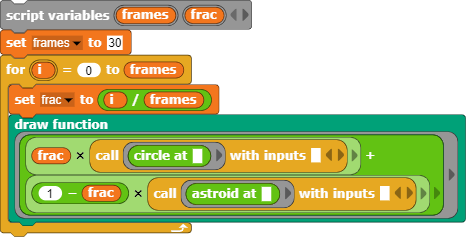Other options:

• using standard library "Iteration, composition".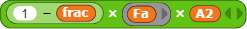can be expressed as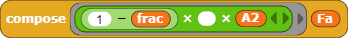The whole expression may be quite complex ... so custom block can be created (variadic compose)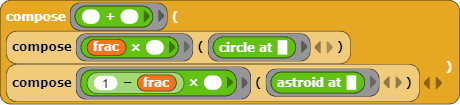• metaprogramming, transform the expression to call the function based on parsed execution tree.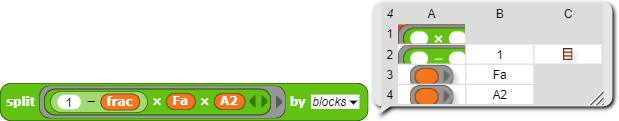• do not draw the "function". Calculate the vector of the drawing then draw a polygon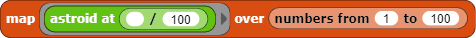With the hyper blocks any manipulation become trivial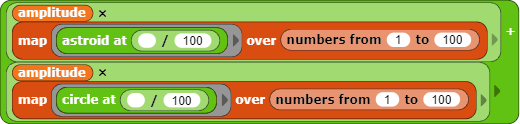• convince theteam to make the "lambda'nes" transitive and the "call" recursive.

Sorry, I don't understand what you're asking for. Could you spell it out and/or give an example? Tnx.

Hello Dardoro,

your first suggestion above gave me the hint to solve my problem.
I built a simple test case: The function feed has a lambda with one input slot as parameter: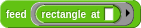feed looks like this: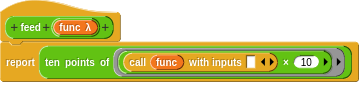By passing the lambda function again with an empty input slot of the call reporter to the function "ten points of" anything works as desired.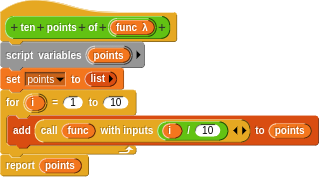I think the problem was a "knot in my brain".
thank you,
Karl

I mean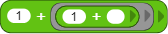become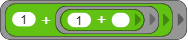and be evaluated properly with the "call".
Or for the practical reason expression can be inlined at the initial evaluation as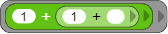The function composition can be expressed just asThank you for helping me as well